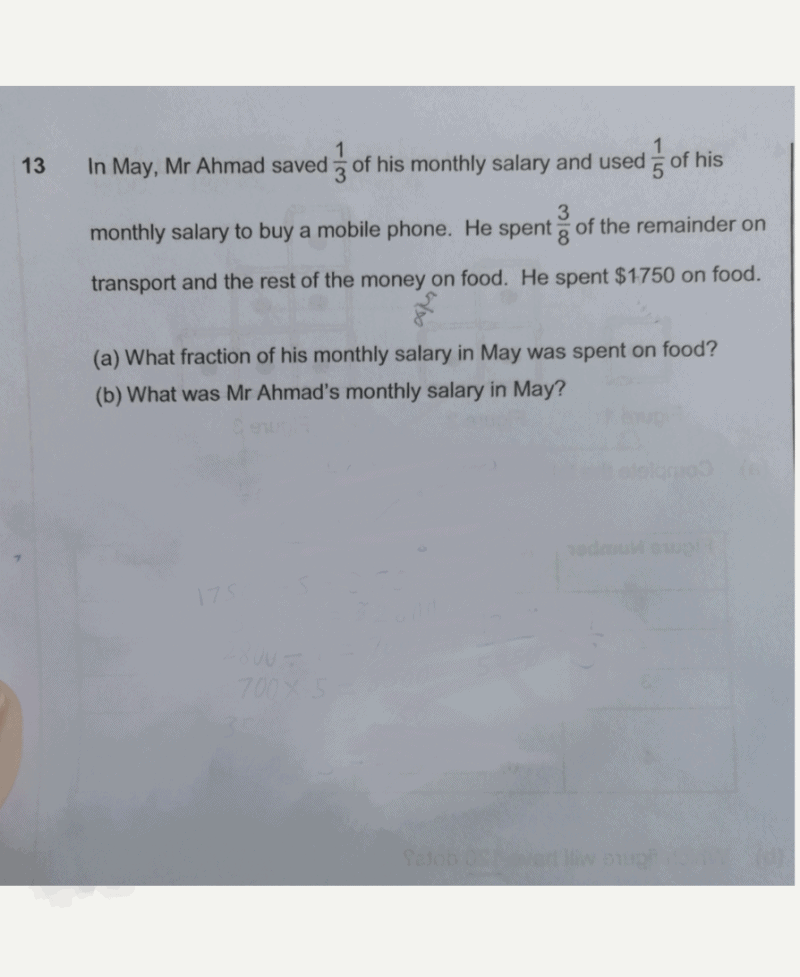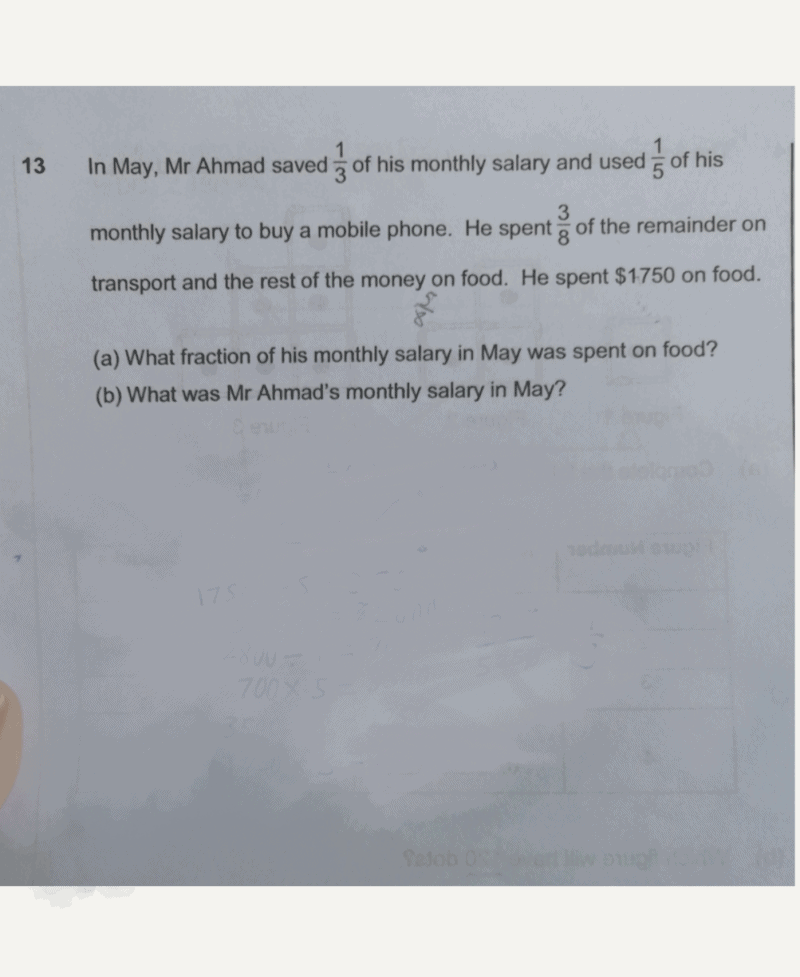# QuestionFirst, we need to find how much of Mr Ahmad’s salary is left after saving and buying a mobile phone, which is 7/15.

Then, we need to find how much was spent on transportation, which is 7/40.

After this, we can now find out a), and the answer is 7/24.

After finding a), we now find a common multiple of 15,8,24. That happens to be 120 for me,and the fraction now is 35/120.

To find the salary, we now need to find how much is 120/120, which is, \$6000. Here, we have found b).

0 Replies 0 Likes